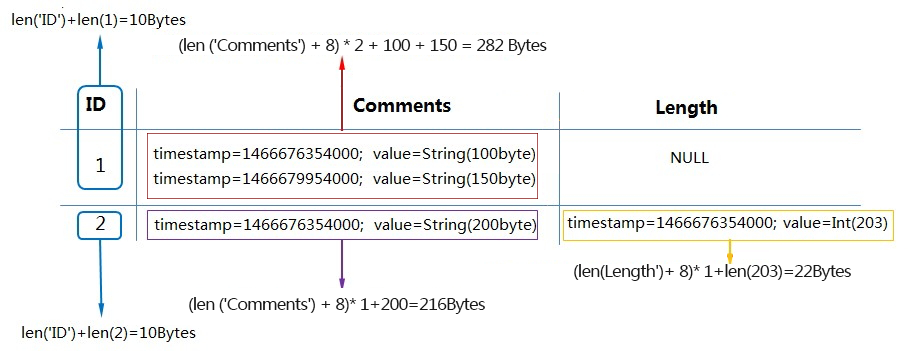Tablestore charges storage usage on an hourly basis based on the total size of data. Tablestore calculates the total size of data based on predetermined intervals and calculates the average data size per hour.

The following section describes how to calculate the size of a single row and tables.

## Calculate the size of a single row

Each row of data in Tablestore uses storage space. After max versions or time to live (TTL) is configured, data of each version includes the version number (8 bytes), column name, and data value.

The size of a single row is calculated based on the formula: ```Size of a single row = Size of primary key columns + Size of all attribute columns. For more information, see Primary keys and attributes.```

The sizes of primary key columns and all attribute columns are calculated based on the formulas:

• Size of primary key columns = Length of all primary key column names + Size of values in primary key columns

• For more information about the size of all attribute columns, see the examples on how to calculate the size of a single row and a table in this topic.

The following table describes how to calculate the size of values.

Date type Number of bytes
STRING The number of bytes used by characters encoded in UTF-8. Tablestore allows empty strings. The size of empty strings is 0.
INTEGER 8.
DOUBLE 8.
BOOLEAN 1.
BINARY The number of bytes used by binary data.

Example: Calculate the size of a row.

The primary column name is ID. Other columns are attribute columns.

1. timestamp = 1466676354000, value = 'zhangsan' timestamp = 1466676354000, value = 20

timestamp = 1466676354000, value = String (100 Bytes)

timestamp = 1466679954000, value = String (150 Bytes)

In the row, two versions are available for the value in the Comments column.

• When MaxVersions is set to 2 and TTL is set to 2592000:
The size of an attribute column is calculated based on the formula: Size of an attribute column = (Length of the attribute column name + 8) × Number of valid versions + Total size of values in the attribute column.
Note When max versions is set to a value greater than 1 or `TTL is set to -1`, each version number uses 8 bytes. The version number is represented by a timestamp. For more information, see Data versions and time to live.
The size of the row is the sum of 10, 20, 22, and 282. Details:
• Size of the primary key column: `len ('ID') + len (1) = 10 bytes`
• Size of the Name attribute column: `(len (‘Name’) + 8) * 1 + len (‘zhangsan’) = 20 bytes`
• Size of the Length column: `(len ('Length') + 8) * 1 + len (20) = 22 bytes`
• Size of the Comments column: `(len ('Comments') + 8) * 2 + 100 + 150 = 282 bytes`
• When MaxVersions is set to 1 and TTL is set to -1:

The size of an attribute column is calculated based on the formula: Size of an attribute column = Length of the attribute column name + Total size of the value in the attribute column.

Note
• When MaxVersions is set to 1 and TTL is set to -1, the version number consumes no bytes.
• Although the value in the Comments column has two versions, only the latest version is calculated because MaxVersions is set to 1.

The size of the row is the sum of 10, 12, 14, and 158, which equals 194 bytes. Details:

• Size of the primary key column: `len ('ID') + len (1) = 10 bytes`
• Size of the Name attribute column: `len ('Name') + len ('zhangsan') = 12 bytes`
• Size of the Length attribute column: `len ('Length') + len (20) = 14 bytes`
• Size of the Comments attribute column: `len ('Comments') + 150 (bytes) = 158 bytes`

## Calculate the size of a table

The size of a table consists of sizes of all rows of data in the table. Example: A table contains the ID primary key column and attribute columns. When MaxVersions is set to 2 and TTL is set to -1: The following figure shows how to calculate the size of the table.• The size of the row whose value in the ID column is 1 = 10 (size of the primary key column) + 282 (size of the values of the two versions in the Comments column). In total, the size of the row whose value in the ID column is 1 is 292 bytes.
• The size of the row whose value in the ID column is 2 = 10 (size of the primary key column) + 216 (size of the value in the Comments column) + 22 (size of the value in the Length column). In total, the size of the row whose value in the ID column is 2 is 248 bytes.
• The size of the table is the sum of 292 and 248, which equals 540 bytes.

If the size of the data in the table remains unchanged within one hour, Tablestore charges storage fees based on 540 bytes. Tablestore does not impose limits on the size of data that can be stored in a table. Tablestore charges storage fees for actual data stored in the table.

Note Tablestore asynchronously deletes expired data in each partition and the additional versions when the max versions value is exceeded. The time used to delete data is related to the total size of the data, which is within 24 hours. Data written to a partition after a data delete operation is performed is charged after the next data delete operation is performed.